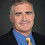## Monday, March 24, 2003

### Foundations of Complexity Lesson 16: Ladner's Theorem

Previous Lesson | Next Lesson

In the 1950's, Friedberg and Muchnik independently showed that there were sets that were computably enumerable, not computable and not complete. Does a similar result hold for complexity theory?

Suppose P≠NP. We have problems that are in P and problems that are NP-complete and we know these sets are disjoint. Is there anything else in NP? In 1975, Ladner showed the answer is yes.

Theorem (Ladner) If P≠NP then there is a set A in NP such that A is not in P and A is not NP-complete.

I wrote up two proofs of this result, one based on Ladner's proof and one based on a proof of Impagliazzo. The write-up is taken mostly from a paper by Rod Downey and myself.

1.Dear Lance, thank you for the writeup which I found the most suitable one I found. A minor remark: the f(n) at the end of the fourth last line of the first proof should be n, right? Martin (Hofmann)

2.Another comment: Muchnik Friedberg is about Turing reductions. So maybe the analogue would rather be: If P =/= PSPACE then there exist languages L1,L2:PSPACE such that L1 not in P^L2 and L2 not in P^L1.Does that hold?

3.replace PSPACE with Delta_2 or something...

4.I wonder if there is a problem with the "first proof": In case 2 on page 2 we must check whether f_i(x) is in SAT or not. This takes time O(2^(|x|^i)) = O(2^(log n)^i) and even for i=2 this is not polynomial in n. Am I wrong?

5.Thanks for your comments on the paper. We can fix the proof by taking say |x|<=log log n. I'll try to update the paper when I get a chance. Basically the same proof works for polytime Turing reductions as well even for NP.

6.Yes, after looking up Arora and Barak, I found the same fix. Thanks very much, this saves me from having to prepare a completely new proof for my class :-)

7.Re Muchnik Friedberg. Somewhat oddly, the proof of Ladner's theorem is actually easier than Muchnik Friedberg because no prioirities are needed. Maybe that's because for small inputs you can solve SAT in polytime but for no inputs however small can one in general solve the halting problem (which plays the role of SAT in Muchnik Friedberg). Usually, complexity is more complicated than computabilitiy, but in this case it seems to be the other way...